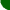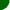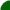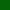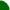welcome/ java-mcmc/ software/ papers/ links/ email mesitemap software essays mathematics unix links

A random field is simply another name for a random function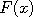, whereranges over some state space. As described in this other coupling demonstration, we can build Markov chains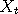by simply chaining together a series of independent random functions: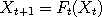for= 0, 1,2, 3 etc. Each such function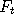has the same fixed probabilities of taking a particular value, i.e. there exists a transition kernel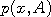such that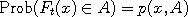Suppose now that we modify the functionsin such a way as to keep these probabilities intact, then by chaining those new functions which we now call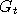say, we obtain a Markov chain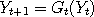which is statistically the same asbut may have other new properties. Whenever we want to make several chains, all of which have the same transition kernel, meet over time it makes sense to modify the original, replacing them with suitably chosenwhich are designed to facilitate coalescence. Perfect simulation requires such constructions, for example. See also here.

Suppose that we draw a graph of the functions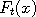and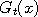. Since these functions are random, the graphs will not always look the same, but certain qualitative features may still stand out. For example, the graphs may be flat or horizontal in some places. In that case the locationswhich belong to that region would all map to the same value, that is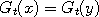for allandwithin the region where the graph ofis flat. Ifwas used to drive two Markov chainsand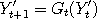say, and if both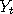and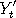both happen to be inside this region, then we would have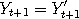afterwards and forever. The Markov chains have coupled. Obviously, the chance of coupling two random chains (which both use the same series) increases the more flat areas exist in each.

To view this applet, please install the Java Plugin.

If you press the button above, a separate window should open demonstrating this scheme in the context of Markov chain Monte Carlo simulation. Starting with a random functionwhich is not necessarily flat anywhere, but has a given set of transition probabilities, we generate successive modifications of this function by flattening randomly chosen regions in such a way that we do not destroy these probabilities. The exact details are described in an accompanying paper (ps/pdf). Eventually, we end up with a `fully flattened' functionto replace.

The controls of this applet are similar to those for the Metropolis-Hastings applet. The main window under the target distribution contains the successive modifications of the random fieldwhose transition probabilities correspond to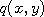, the proposal distribution for a Metropolis-Hastings algorithm. The third and fourth panels on the left represent histograms of respectively the modified and unmodified random functions (also called fields here) after metropolization. If you wait long enough, both histograms will look alike, as they should since the transition probabilities have been preserved.

 sitemap software essays mathematics unix links